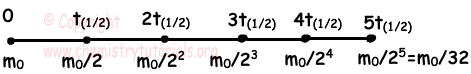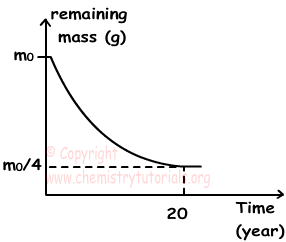## Half Life and Radioactive Decay Rates

Half Life and Radioactive Decay Rates

Half Life: Unstable nucleus does radioactive decays and decrease its mass. Half time is time required for half of mass of radioactive matter to decay. It is depends on types of matter or n/p ratio. If initial mass of matter is m0, after t time it has mass m, and if half life of matter is t(1/2);

when t=t(1/2) m=m0/2

Picture given below shows amount of mass as the time passes;After first half time, mass decreases to m0/2, after second half time it decreases half of its previous value. If we write it as equation we get;

m=m0/2n

Where "n" is the number of half life,

n=t/t(1/2)

Now we draw graph of mass vs. time of radioactive decay.As you can see from the graph, mass decreases with time but never becomes zero.

Some properties of half life:

• Every different nucleus has its own half life, in other words half life is specific properties of nucleus. On the other hand isotopes of atoms also have different half life since their number of neutrons are different.
• Half life does not depend on initial mass of matter, it is constant for each nucleus.
• Half life does not depend on temperature and pressure.

Example: If time passes for decay 31/32 of matter is 60 years, find its half life.

Solution:

Since 31/32 of matter decays, 1/32 of matter is left. If initial mass is m0 ;As you can see from the picture, there are 5 half life;

5t(1/2)=60 years

t(1/2)=12 years.

Example: Which ones of the following statements are true for half life of matters;

I. It changes with the change in mass of matter.

II. It changes with changes in the temperature.

III. It changes with the changes of types of matters.

Solution:

As we mention before, half life of matter is only depends on types of matter. III is true.

Rate of Decay:

Rate of decay is number of disintegrated nucleus in unit time. Rate of decay depends on half life and mass of matter.

• Rate of decay is inversely proportional to half life of matter. If masses of two matters are equal than matter having smaller half life has higher rate of decay.
• Rate of decay is directly proportional to mass of radioactive matter.
• Since mass of matter decreases in decay process, rate of decay also decreases with time.

Relation between half life and rate of decay is;

k=0,693/t(1/2)

where k is rate of decay and t(1/2) is half life.

Example: Graph given below shows radioactive decay of matter X.Use this graph and state whether the following statements are true or false;

I. Half life of matter is 10 years.

II. Rate decay of matter after 20 years is smaller than initial rate decay.

III. Amount of matters decay in first half decay and second half decay are equal.

Solution:

I. When mass decreases from m0 to m0/4, it does two half life;

2t(1/2)=20

t(1/2)=10 years I is true.

II. Since the mass after second decay is smaller than initial mass and rate of decay is directly proportional to mass, rate of decay after second decay is smaller than initial value. II is true.

III. Decrease in mass in first decay;

m0-m0/2=m0/2 g

Decrease in mass after second decay;

m0/2-m0/4=m0/4 g

Thus, decrease in masses are not equal in first and second decay. III is false.

Nuclear Chemistry (Radioactivity) Exams and  Problem Solutions

Author:

© Copyright www.ChemistryTutorials.org, Reproduction in electronic and written form is expressly forbidden without written permission of www.ChemistryTutorials.org. Privacy Policy